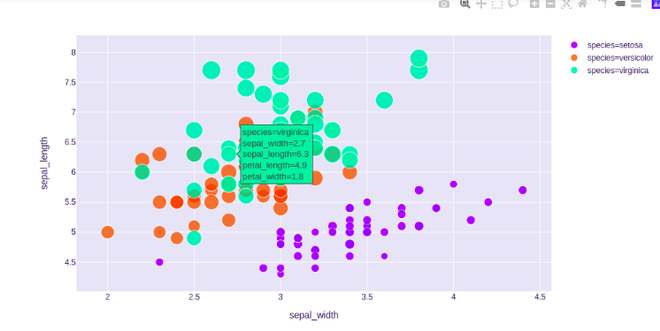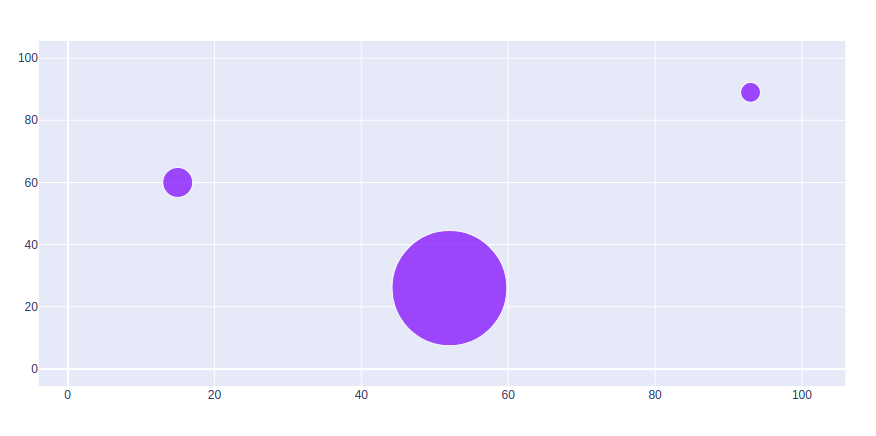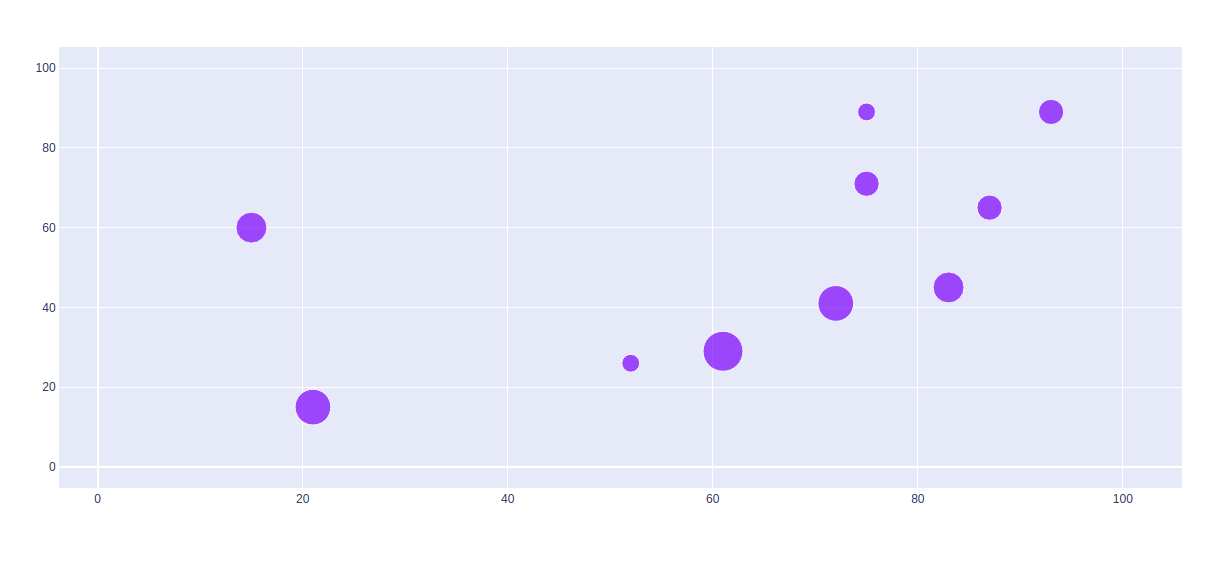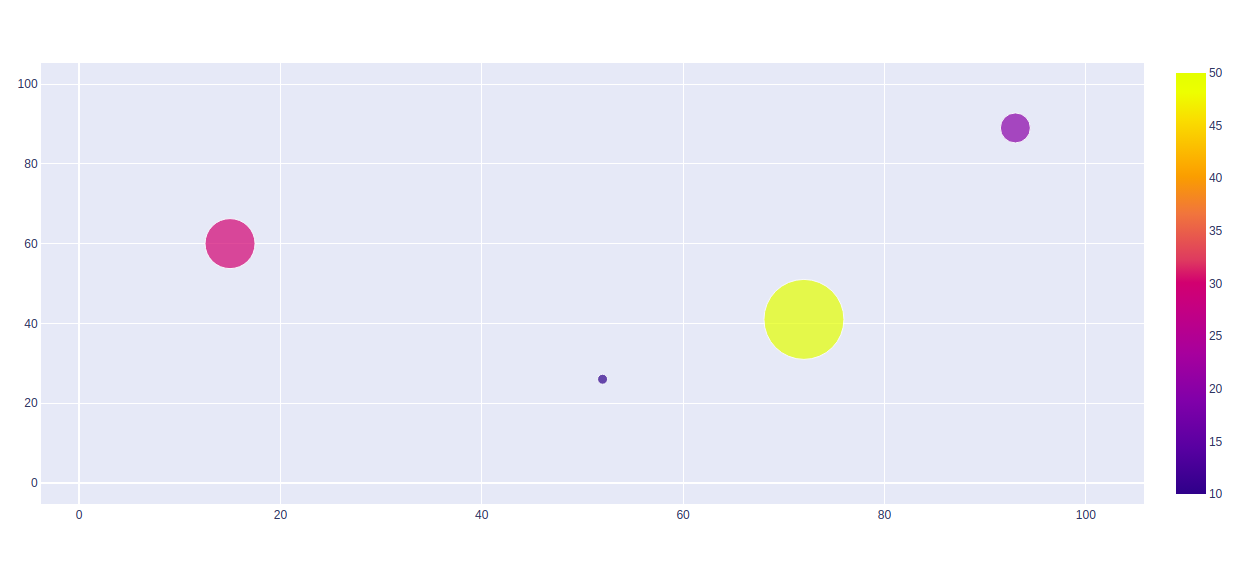# Bubble chart using Plotly in Python

• Last Updated : 03 Jul, 2020

Plotly is a Python library which is used to design graphs, especially interactive graphs. It can plot various graphs and charts like histogram, barplot, boxplot, spreadplot, and many more. It is mainly used in data analysis as well as financial analysis. Plotly is an interactive visualization library.

## Bubble Chart

The bubble chart in Plotly is created using the scatter plot. It can be created using the scatter() method of `plotly.express`. A bubble chart is a data visualization which helps to displays multiple circles (bubbles) in a two-dimensional plot as same in scatter plot. A bubble chart is primarily used to depict and show relationships between numeric variables.

Example:

## Python3

 `import` `plotly.express as px ` ` `  `df ``=` `px.data.iris() ` ` `  `fig ``=` `px.scatter(df, x``=``"sepal_width"``, y``=``"sepal_length"``, ` `                 ``color``=``"species"``, ` `                 ``size``=``'petal_length'``,  ` `                 ``hover_data``=``[``'petal_width'``]) ` ` `  `fig.show()`

Output:## Set Marker Size

Marker size and color are used to control the overall size of the marker. Marker size helps to maintain the color inside the bubble in the graph. Scatter is used to actually scale the marker sizes and color based on data.

Example:

## Python3

 `import` `plotly.graph_objects as px ` `import` `numpy as np ` ` `  ` `  `# creating random data through randomint  ` `# function of numpy.random  ` `np.random.seed(``42``) ` ` `  `random_x``=` `np.random.randint(``1``,``101``,``100``)  ` `random_y``=` `np.random.randint(``1``,``101``,``100``) ` ` `  `plot ``=` `px.Figure(data``=``[px.Scatter( ` `    ``x ``=` `random_x, ` `    ``y ``=` `random_y, ` `    ``mode ``=` `'markers'``, ` `    ``marker_size ``=` `[``115``, ``20``, ``30``]) ` `]) ` `                  `  `plot.show()`

Output:## Scaling the Size of Bubble Charts

To scale the bubble size,use the parameter sizeref. To calculate the values of sizeref use:

sizeref = 2. * max(array of size values) / (desired maximum marker size ** 2)

Example:

## Python3

 `import` `plotly.graph_objects as px ` `import` `numpy as np ` ` `  ` `  `# creating random data through randomint  ` `# function of numpy.random  ` `np.random.seed(``42``) ` ` `  `random_x``=` `np.random.randint(``1``,``101``,``100``)  ` `random_y``=` `np.random.randint(``1``,``101``,``100``) ` ` `  `size ``=` `[``20``, ``40``, ``60``, ``80``, ``100``, ``80``, ``60``, ``40``, ``20``, ``40``] ` ` `  `plot ``=` `px.Figure(data``=``[px.Scatter( ` `    ``x ``=` `random_x, ` `    ``y ``=` `random_y, ` `    ``mode ``=` `'markers'``, ` `    ``marker``=``dict``( ` `        ``size``=``size, ` `        ``sizemode``=``'area'``, ` `        ``sizeref``=``2.``*``max``(size)``/``(``40.``*``*``2``), ` `        ``sizemin``=``4` `    ``) ` `)]) ` `                  `  `plot.show()`

Output:## Showing Color scale

The color scale is a specialized label which helps to displays a color map with its scale, the color scale is used to display a color palette and its numerical scale for color mapped.

Example:

## Python3

 `import` `plotly.graph_objects as px ` `import` `numpy as np ` ` `  ` `  `# creating random data through randomint  ` `# function of numpy.random  ` `np.random.seed(``42``) ` ` `  `random_x``=` `np.random.randint(``1``,``101``,``100``)  ` `random_y``=` `np.random.randint(``1``,``101``,``100``) ` ` `  `plot ``=` `px.Figure(data``=``[px.Scatter( ` `    ``x ``=` `random_x, ` `    ``y ``=` `random_y, ` `    ``mode ``=` `'markers'``, ` `     ``marker``=``dict``( ` `       ``color ``=` `[``10``, ``20``, ``30``, ``50``],  ` `       ``size ``=` `[``10``, ``30``, ``50``, ``80``], ` `       ``showscale``=``True` `     ``) ` `)]) ` `                  `  `plot.show()`

Output:My Personal Notes arrow_drop_up
Recommended Articles
Page :